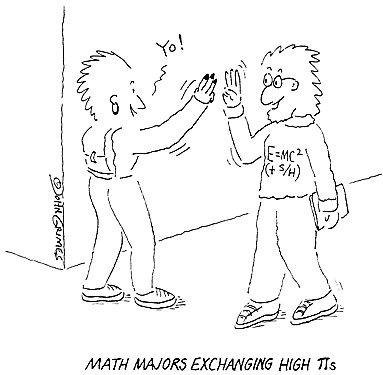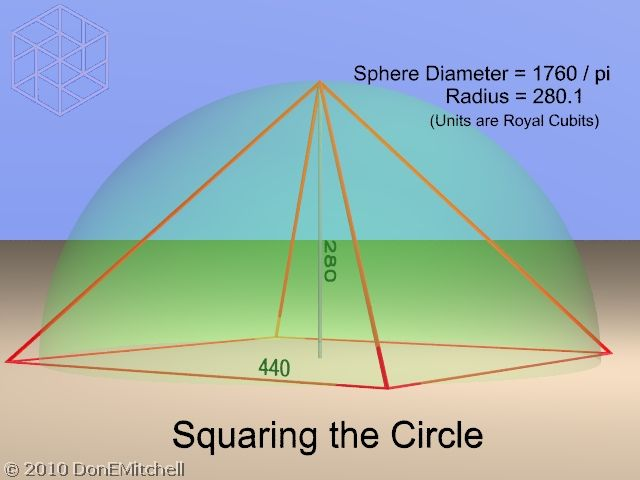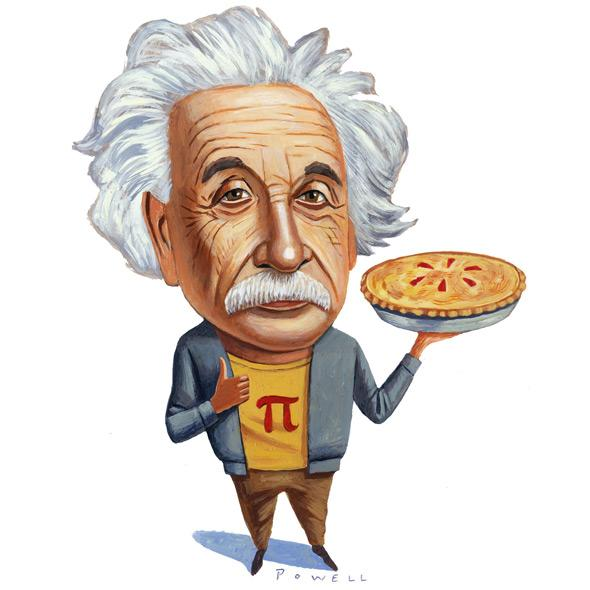## 36 Surprising Facts About PiPi is the most studied number in mathematics. And that is for a good reason. The number pi is an integral part of many incredible creations including the Pyramids of Giza. Yes, that’s right.

### Here are 36 facts you will love about pi.

1. The symbol for Pi has been in use for over 250 years. The symbol was introduced by William Jones, an Anglo-Welsh philologist in 1706 and made popular by the mathematician Leonhard Euler.

2. Since the exact value of pi can never be calculated, we can never find the accurate area or circumference of a circle.

3. March 14 or 3/14 is celebrated as pi day because of the first 3.14 are the first digits of pi. Many math nerds around the world love celebrating this infinitely long, never-ending number.

4. The record for reciting the most number of decimal places of Pi was achieved by Rajveer Meena at VIT University, Vellore, India on 21 March 2015. He was able to recite 70,000 decimal places. To maintain the sanctity of the record, Rajveer wore a blindfold throughout the duration of his recall, which took an astonishing 10 hours!

Can’t believe it? Well, here is the evidence:

5. If you aren’t a math geek, you would be surprised to know that we can’t find the true value of pi. This is because it is an irrational number. But this makes it an interesting number as mathematicians can express π as sequences and algorithms.6. Pi is just another weird or boring mathematical number. It is a part of Egyptian mythology. People in Egypt believed that the pyramids of Giza were built on the principles of pi. The vertical height of the pyramids have the same relationship with the perimeter of their base as is the relationship between a circle’s radius and its circumference. The pyramids are phenomenal structures in themselves being one of the seven wonders of the world and attract tourists. So having π as the core principle makes it really special for architects.

7. Although Pi day is celebrated on March 14 (3/14), the exact time for celebration is 1:59 pm so that the exact number 3.14159 can be reached.

8. Physicist Larry Shaw started 14 March as Pi day at San Francisco’s Exploratorium. There he is known as the Prince of Pi.

9. There is an entire language made on the number Pi. But how is that possible? Well, some people love pi enough to invent a dialect in which the number of letters in the successive words are the same as the digits of pi. But it is not just another nerd quirk that nobody knows about. Mike Keith wrote an entire book, called ‘Not a Wake’ in this language.

10. There are many records that show that pi was discovered a long time ago in the The Babylonians knew of pi approximately 4000 years ago. Evidence shows that Babylonians calculated pi as 3.125.King James Bible also gives an approx value of pi as the length of the forearm from the elbow to the middle finger tip.

11. There is an interesting reason why the name ‘pi’ was coined. Before the name pi came, mathematicians had to say a mouthful. The only descriptive phrase they could use was “the quantity which when the diameter is multiplied by it, yields the circumference”. Pi was named pi by William Jones, a not-so-popular mathematician.

12. The number of digits in the number pi is a phenomenon in itself. Humans can never find all the digits of number pi because of its very definition. Babylonian civilization used the fraction 3 ⅛, the Chinese used the integer 3. By 1665, Isaac Newton calculated pi to 16 decimal places. This was before the computers were invented, so determining 16 digits was a big deal. It was in the early 1700s that Thomas Lagney calculated 127 decimal places of pi reaching a new record. In the second half of the twentieth century, the number of digits of pi increased from about 2000 to 500,000 on the CDC 600. But this record was broken to a whole new level in 2017 when a Swiss scientist computed more than 22 trillion digits of pi which took more than a hundred days.

13. Pi is considered divine. No, not in the literal sense. The number is ‘transcendental’ in mathematical terms. A mathematician, Johann Lambert, gave proof that pi is irrational by giving the tangent of x using continued fraction.

14. Usefulness of pi has been a matter of debate although it is loved by a lot of math lovers. Some believe that tau (which amounts to 2π) is a better suited and intuitive irrational number. For instance, you can multiply tau with radius and calculate the circumference of a circle more intuitively. Tau/4 also represents the angle of a quarter of a circle. Hence its intuitiveness makes it more appealing to some math enthusiasts.

15. In the Exploratorium science museum, a circular parade happens every year on pi day. Each person participating holds one of the digits in the number pi. It wasn’t celebrated around the United States like it is done now until the Congress passed Resolution 224. The purpose of celebrating Pi day was to cultivate a higher level of enthusiasm for math and science.

16. In Carl Sagan’s novel Contact, scientists manage to dig deep into the mystery of the number pi to uncover the hidden messages from the creator of the human race. This new wisdom is capable of bringing depth to our consciousness.

17. The film Pi: Finding Faith in Chaos depicts the efforts of the protagonist in searching for answers about pi and, in turn, the universe. This search drives him nuts. But the good part is that this movie won the Director’s Award at the Sundance film festival.

18. A crop circle was found in 2008 that showed a coded image containing the first ten digits of pi.19. The calculation of pi is a stress test for a computer. It works just like a digital cardiogram since it indicates the level of activity within the computer’s processor.

20. Givenchy sells a men’s cologne with the name ‘Pi’. The company markets this product as capable of enhancing sexual appeal of intelligent and visionary men.

21. The number Pi is not just an important part of conversations among mathematicians or students. In the famous O.J. Simpson trial, the defense attorney and FBI agent’s argument revolved around the value of pi. This argument over pi showed that the FBI agent’s findings in the case weren’t accurate because he used pi inaccurately.

22. The number pi was so mysterious that a Dutch-German mathematician, Ludolph van Ceulen, spent most of his life calculating the first 36 digits of pi. It is said that the first 36 numbers were engraved on his tombstone, which is now lost.

23. William Shanks, a British mathematician, worked manually to find the digits of pi. He spent many years trying to calculate the pi digits by hand and found the first 707 digits. Unfortunately, the 527th digit he found was wrong, which made his efforts of finding the remaining digits useless because they were all wrong by default.

24. Pi has a sacred bond with the circle. A circle’s angle spans 360 degrees around its centre and it is a coincidence that the number 360 is at the 359th digit position of pi.

25. In the year 1888, an Indiana country doctor claimed that he learnt the exact measure of a circle through supernatural means. He believed in his “supernatural” knowledge so much that he filed a proposal to pass a bill in the Indiana legislature so that he could copyright his genius findings. However, there was a math professor in the legislature who showed the fellow how his proposed bill will result in a wrong value of pi.

26. Even comedians use pi to make people crack at their jokes. John Evans, a comedian, once said in his performances, “What do you get if you divide the circumference of a jack-o’-lantern by its diameter? Pumpkin π.”27. The number pi is literally infinitely long. But the number 123456 doesn’t appear anywhere in the first million digits of pi. It is a bit shocking because if a million digits of pi don’t have the sequence 124356, it definitely is the most unique number.

28. Why are we so obsessed about pi? Because we are looking for a pattern. Human beings love to find analogies and patterns in everything. And the number pi is so long and mysterious that mathematicians love to find patterns in this number.

29. Chinese people were far ahead of the West in finding the digits of pi. Why? As many mathematicians believe, the Chinese language is more conducive to mathematical computations. Chinese mathematicians were ahead in the pi game because of two reasons: they had decimal notations and they had a symbol for the number zero. It wasn’t until the late middle ages that European mathematicians started using the number zero. At that time, European mathematicians partnered with Arab and Indian minds to bring the symbol of zero into their system.

30. In the ancient times, mathematicians used a unique method to calculate pi. They would add more and more sides to a polygon so that its area approached the area of a circle. Archimedes, the most famous Greek mathematician and inventor, used a polygon with 96 sides. Many other mathematicians also used this polygon-method to compute the infinitely long number pi. In China, a mathematician used about 200 and then over 3,000 sides in a polygon to arrive at the value 3.14159. Some other used about 25,000 sides to calculate pi. It is quite clear how obsessed the mathematicians were with the number pi.

31. The usage of pi has evolved over the years. Before 17th century, pi was only used for circles, as we mentioned earlier how strong the bond between pi and a circle is. But in the 17th century, people realized that pi can be used to calculate areas of other curves including arches and hypocycloids. In the 20th century, pi was used for a wide set of applications in areas such as probability and various mathematical theories.

32. Many mathematicians believe that it is more accurate to say that a circle has infinite corners than it is to say that it has none. It is only reasonable to assume that this “infinite” number of corners correlates to the infinite number of digits of pi.33. The number pi is hard to calculate but is super effective when you use it to calculate other things. For instance, if you round the number pi to just 9 digits after the decimal and use it to calculate earth’s circumference, the results would be amazingly accurate. For every 25,000 miles, the number pi will only err to 1/4th of an inch. If you love math, you will realize how amazing this actually is.

34. Pi is so amazing and “mysterious” that it has been used in mysterious situations in movies. In the 1996 thriller movie Torn Curtain, Pi is the secret code.

35. People are forever going crazy about calculating the most number of digits of pi. It is like a competition that never ends. In the year 2010, a Japanese engineer and an American computer wizard broke the record for the most number of pi digits by calculating upto 5 trillion digits. The amazing part is that they didn’t use any supercomputers. They just used desktop computers, 20 external hard disks and used their brilliant minds.

36. There is a Star Trek episode called ‘Wolf in the Fold’ in which Spock thwarts the evil computer by challenging it to compute to the last digit of pi. It is amazing how no evil movie character can do anything about the number pi.

37. The Greek letter π is the first letter of the word periphery and perimeter. And as we all know, pi is the ratio of a circle’s “periphery” to its diameter.

38. Many genius minds have a relation with the Pi day. Albert Einstein was born on Pi day. Stephen Hawkings died on 3/14, Pi day at the age of 76.